# Chemical Reactions and Equations Class 10 Extra Questions with Answers Science Chapter 1

In this page, we are providing Chemical Reactions and Equations Class 10 Extra Questions and Answers Science Chapter 1 pdf download. NCERT Extra Questions for Class 10 Science Chapter 1 Chemical Reactions and Equations with Answers will help to score more marks in your CBSE Board Exams.

## Class 10 Science Chapter 1 Extra Questions and Answers Chemical Reactions and Equations

Extra Questions for Class 10 Science Chapter 1 Chemical Reactions and Equations with Answers Solutions

### Extra Questions for Class 10 Science Chapter 1 Very Short Answer Type

Question 1.
How does the food become rancid?
Food becomes rancid when fat and oils present in the food are oxidised.

Question 2.
A student burnt a metal A found in the form of ribbon. The ribbon burnt with a dazzling flame and a white powder B was formed which was basic in nature. Identify A and B. Write the balanced chemical equation.
X = Mg,  Y = MgO,  Mg + O2 → 2MgO

Question 3.
What is a balanced chemical equation?
An equation that has equal number of atoms of each element on both the sides of the equation is called a balanced chemical equation, i.e., mass of the reactants is equal to mass of the products.
For example, $$2 \mathrm{Mg}+\mathrm{O}_{2} \stackrel{\Delta}{\longrightarrow} 2 \mathrm{MgO}$$

Question 4.
Write a balanced equation for a chemical reaction that can be characterised as precipitation.
BaCl2(aq) + Na2SO4 (aq) → BaSO4(s) + 2NaCl(aq)

Question 5.
What is rust?
It is a brown mass known as hydrated ferric oxide. Its formula is Fe2O3. xH2O.

Question 6.
A zinc rod is left for nearly 20 minutes in a copper sulphate solution. What change would you observe in the zinc rod?
The zinc rod will change into zinc sulphate.

Question 7.
Name two salts that are used in black and white photography.
Both silver chloride and silver bromide are used in black and white photography.

Question 8.
Which chemical process is used for obtaining a metal from its oxide?
The process is known as the reduction of metal oxide.

Question 9.
If you collect silver coins and copper coins you may have seen that after some days a black coating forms on silver coins and a green coating on copper coins. Which chemical phenomenon is responsible for these coatings? Write the chemical name of the black and green coatings.
Corrosion is responsible for the formation of this coating. Black coating is due to formation of Ag2S and green coating is due to formation of CuCO3.Cu(OH)2.

Question 10.
When carbon dioxide is passed through lime water, it turns milky, why?
Lime water (calcium hydroxide) combines with carbon dioxide to form a suspension of calcium carbonate which makes lime water milky.
Ca(OH)2 + CO2 → CaCO3 + H2O

Question 11.
Identify the most reactive and least reactive metal: Al, K, Ca, Au.
Most reactive metal: K(Potassium); least reactive metal: Au(gold).

Question 12.
X + Y SO4 → X SO4 + Y
Y + X SO4 → No reaction
Of the two elements T and Y which is more reactive and why?
‘X’ is more reactive than ‘Y since it has displaced ‘Y’ in the displacement reaction.

Question 13.
Why is it necessary to balance a chemical equation?
An equation is balanced in order to satisfy the law of conservation of mass according to which total mass of the reactants is equal to the total mass of the products, i.e., mass can neither be created nor be destroyed during any chemical change.

Question 14.
During electrolysis of water, the gas collected in one test tube is double than the other, why?
On electrolysis, water decomposes into hydrogen and oxygen in the ratio 2 : 1 by volume so, H2 gas collected in one test tube is double than O2.

Question 15.
Represent decomposition of ferrous sulphate with the help of balanced chemical equation.
2FeSO4 (s) → Fe2O3 (s) + SO2 (g) + SO3 (g)

Question 16.
What is a chemical equation?
A chemical equation is a symbolic notation that uses formulae instead of words to represent a chemical equation.

Question 17.
A teacher took a few crystals of sugar in a dry test tube and heated the test tube over a flame. The colour of sugar turned black. Explain why?
Sugar is a complex compound which on heating undergoes decomposition. Water gets evaporated thereby leaving behind black carbon in the test tube.

Question 18.
Name two metals which do not get corroded.
Gold (Au) and platinum (Pt) do not get corroded.

Question 19.
Identify the compound oxidised in the following reaction:
H2S (g) + Cl2 → S(s) + 2HCl (g)
H2S is oxidised.

Question 20.
Why is a magnesium ribbon cleaned before burning?
Magnesium reacts with moist air and forms a layer of oxide, MgO (white), on its surface. So, a magnesium ribbon is cleaned to remove the oxide layer before burning.

Question 21.
State the chemical change that takes place when limestone is heated.
Calcium carbonate decomposes on heating to give calcium oxide and carbon dioxide.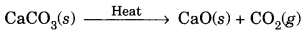Question 22.
On which chemical law, balancing of chemical equation is based?
Balancing of a chemical equation is based on the law of conservation of mass.

Question 23.
Name the term used for the solution of the reactants or products when dissolved in water.
Aqueous

Question 24.
What happens when magnesium ribbon burns in air?
Ans. When magnesium ribbon burns in air, it combines with the oxygen to form magnesium oxide.
2Mg(s) + O2(g) → 2MgO(s)

Question 25.
Give an example of an exothermic reaction.
CH4(g) + 2O2(g) → CO2(g) + 2H2O(g) + Heat (evolved)

Question 26.
What type of reaction is this: Na2SO4 + BaCl2 → BaSO4 + 2NaCl
It is a double displacement reaction.

Question 27.
Give an example of exothermic reaction.
N2 (g) + O2 (g) → 2NO (g) – Heat (absorbed)

Question 28.
A substance X used for coating iron articles is added to a blue solution of a reddish brown metal Y. The colour of the solution gets discharged. Identify X and Y and also the type of reaction.
X = Zn, Y = Cu, Displacement reaction.

Question 29.
Name the gas evolved when zinc reacts with dil. HCl.
Hydrogen gas is evolved.

### Extra Questions for Class 10 Science Chapter 1 Short Answer Type I

Question 1.
You are given the following materials
(i) Marble chips (ii) dilute hydrochloric acid (iii) Zinc granules
Identify the type of reaction when marble chips and zinc granules are added separately to acid taken in two test tubes.
(i) Marble chips react with dilute hydrochloric acid to form calcium chloride and carbon dioxide. It is a double displacement reaction.
CaCO3 + 2HCl → CaCl2 + H2O + CO2

(ii) Zinc granules react with dilute hydrochloric acid to give hydrogen gas. It is a displacement reaction.
Zn (s) + 2HCl → ZnCl2 (aq) + H2 (g)

Question 2.
What do you understand by precipitation reaction? Explain with suitable examples.
The reaction in which two compounds in their aqueous state react to form an insoluble compound. When two reactants react and product formed remains insoluble and settles as a solid it is substance (precipitate) is called a precipitation reaction.

For example,
(i) When aqueous solution of sodium sulphate is mixed with an aqueous solution or barium chloride, barium sulphate is obtained as a white precipitate.
Na2SO4 (aq) + BaCl2 (aq) → BaSO4 (s) + 2NaCl (ag)

(ii) When aqueous solution of sodium chloride is mixed with an aqueous solution of silver nitrate, silver chloride is obtained as a white precipitate.

Question 3.
What happens when aqueous solutions of sodium sulphate and barium chloride are mixed? What type of reaction is it?
On mixing the solutions of sodium sulphate and barium chloride, a white precipitate of barium sulphate is obtained.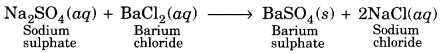It is a double displacement reaction.

Question 4.
Explain the following terms with suitable examples.
(a) Oxidation
(b) Reduction
(a) Oxidation is a process of addition of oxygen to a substance or removal of hydrogen from a substance, for example,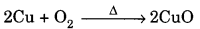Copper is oxidised to CuO, as oxygen is added to copper.

(b) It is the process of removal of oxygen from a substance or addition of hydrogen to a substance, for example,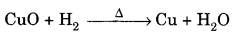Copper oxide is reduced to copper as it involves removal of oxygen.

Question 5.
Complete the missing components/variables given as x and y in the following reactions.  [NCERT Exemplar]
(a) Pb(NO3)2 (aq) + 2Kl (aq) → PbI2 (x) + 2KNO3 (y)
(b) Cu (s) + 2AgNO3 (aq) → Cu(NO3)2 (aq) + x (s)
(c) Zn (s) + H2SO4 (aq) → ZnSO4 (x) + H2 (y)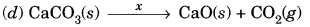(a) x = (s), y = (aq)
(b) x = 2Ag
(c) x = (aq); y = (g)
(d) x = heat

Question 6.
An iron knife kept dipped in a blue copper sulphate solution turns the blue solution light green. Why?
We know that iron is more reactive than copper, so it displaces copper from copper sulphate solution and forms ferrous sulphate which is of light green colour.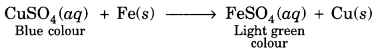Question 7.
A, B and C are three elements which undergo chemical reactions in the following way.
A2O3 + 2B → B2O3 + 2A
3CSO4 + 2B → B2(SO4)3 + 3C
3CO + 2A → A2O3 + 3C
(a) Which element is most reactive?
(b) Which element is least reactive?
(a) The most reactive element is ‘B’. It has displaced both ‘A’ and ‘C’ from their compounds.
(b) The least reactive element is ‘C’ as it has been displaced by both ‘A’ and ‘B’.

Question 8.
Write the balanced chemical equations for the following reactions and identify the type of reaction in each case.
(a) Nitrogen gas is treated with hydrogen gas in the presence of a catalyst at 773 K to form ammonia gas.
(b) Sodium hydroxide solution is treated with acetic acid to form sodium acetate and water.
(c) Ethanol is warmed with ethanoic acid to form ethyl acetate in the presence of concentrated H2SO4.
(d) Ethene is burnt in the presence of oxygen to form carbon dioxide, water and releases heat and light.  [NCERT Exemplar]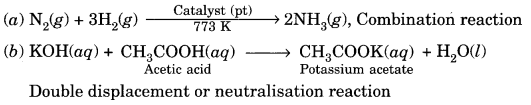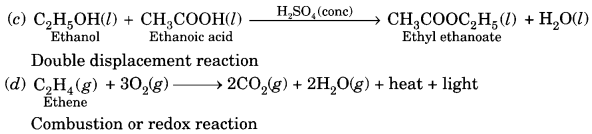Question 9.
What is lime water test for the detection of carbon dioxide?
When carbon dioxide gas is passed through lime water, it turns milky due to the formation of a milky suspension (precipitate) of calcium carbonate.
Ca(OH)2 (aq) + CO2 (g) → CaCO3 (s) + CO2 (g)

Question 10.
During the reaction of some metals with dilute hydrochloric acid, following observations were made,
(a) Silver metal does not show any change.
(b) The temperature of the reaction mixture rises when aluminium (Al) is added.
(c) The reaction of sodium metal is found to be highly explosive.
(d) Some bubbles of a gas are seen when lead (Pb) is reacted with the acid.
Explain these observations giving suitable reasons.   [NCERT Exemplar]
(a) No change takes place because silver metal does not react with hydrochloric acid in normal situations.
(b) The reaction between hydrochloric acid and aluminium is exothermic, thus the temperature of the reaction mixture rises when aluminium is added.
(c) Since, sodium is a highly reactive metal, thus it reacts with hydrochloric acid vigorously and produces a large amount of heat. Thus, the reaction is exothermic.
(d) Bubbles of hydrogen gas are formed when lead react with dilute hydrochloric acid.
Pb + 2HCl → PbCl2 + H2

Question 11.
A copper coin is kept in a solution of silver nitrate for some time. What will happen to the coin and the colour of the solution?
We know that copper is more reactive than silver, so it will displace silver from its salt solution.
Cu (s) + 2AgNO3 (aq) → Cu(NO3)2 (aq) + 2Ag (s)
So, the solution will turn blue due to the formation of copper nitrate.

Question 12.
An aqueous solution of metal nitrate P reacts with sodium bromide solution to form yellow ppt. of compound Q which is used in photography. Q on exposure to sunlight undergoes decomposition reaction to form metal present in P along with reddish brown gas. Identify P and Q. Write the chemical reaction and type of chemical reaction.
P = Ag NO3, Q = AgBr
AgNO3 (aq) + NaBr (aq) → NaNO3 (aq) + AgBr (s); Double decomposition reaction
2AgBr (s) → 2Ag (s) + Br2 (g); Photochemical decomposition reaction

Question 13.
What happens when iron nails are immersed in copper sulphate solution? What type of reaction is it?
When iron nails are immersed in copper sulphate solution, iron ions displace copper ions and a new compound ferrous sulphate is formed.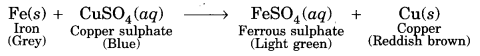It is a diplacement reaction.

Question 14.
Which of the following reaction is possible. Explain giving suitable reason.
(i) Zn (s) + CuSO4 (aq) → ZnSO4 (aq) + Cu (s)
(ii) Fe (s) + ZnSO4 (aq) → FeSO4 (aq) + Zn (s)
(iii) Zn (s) + FeSO4 (s) → ZnSO4 (aq) + Fe (s)
Reaction (i) and (iii) are possible.
(i)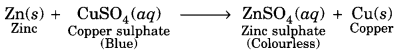Zinc is more reactive than copper, therefore, it can displace copper from copper sulphate solution.

(iii) Zn (s) + FeSO4 (aq) → ZnSO4 (aq) + Fe (s)
Zn is more reactive than Fe, therefore, it can displace iron from ferrous sulphate solution.

Reaction (ii) is not possible as iron is less reactive than zinc, hence, it cannot displace Zn.

Question 15.
Which among the following changes are exothermic or endothermic in nature?
(a) Decomposition of ferrous sulphate
(b) Dilution of sulphuric acid
(c) Dissolution of sodium hydroxide in water
(d) Dissolution of ammonium chloride in water  [NCERT Exemplar]
(a) endothermic
(b) exothermic
(c) exothermic
(d) endothermic.

Question 16.
Advantage. In some metals a protective layer is formed on its surface due to corrosion which prevent it from further corrosion.

Example, Aluminium (Al) forms a layer of aluminium oxide (Al2O3) by corrosion. This layer prevents further corrosion of aluminium.

### Extra Questions for Class 10 Science Chapter 1 Short Answer Type II

Question 1.
What is corrosion? State condiyions necessary for rusting of iron. How is rusting harmful?  [NCERT Exemplar]
Corrosion. The process of eating away of the metal surface by the action of atmospheric reagents like water, oxygen and acids changing the metal into its compound is called corrosion.

Rusting of iron. When iron objects are exposed to atmosphere, they are attacked by air and moisture (water) of the atmosphere and a brown and orange coloured layer is formed on the surface. It is called rust which is mainly hydrated iron (III) oxide Fe2O3. xH2O.

Harmful effect of rusting. Hydrated iron (III) oxide is a brittle substance and falls off from the surface of iron and thus the object is damaged. Holes, cavities and roughness of surfaces are the result of rusting of an iron object.

Conditions necessary for rusting:

• Open surface of the metal
• Presence of air (oxygen)
• Presence of moisture (water).

Question 2.
The gases hydrogen and chlorine do not react with each other even if kept together for a long time. However, in the presence of sunlight, they readily combine. What actually happens?   [NCERT Exemplar]
In chemical reactions, energy is needed to break the bonds present in the reacting molecules so that they may combine to form the products. In this reaction, sunlight is the source of energy in the form of photons. The energy made available by sunlight helps in breaking the bonds and this leads to chemical reaction between hydrogen and chlorine.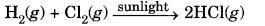Question 3.
Write the balanced chemical equations for the following reactions and identify the type of reaction in each case.
(a) Thermite reaction, iron (III) oxide reacts with aluminium and gives molten iron and aluminium oxide.
(b) Magnesium ribbon is burnt in an atmosphere of nitrogen gas to form solid magnesium nitride.
(c) Chlorine gas is passed in an aqueous potassium iodide solution to form potassium chloride solution and solid iodine.
(d) Ethanol is burnt in air to form carbon dioxide, water and releases heat.   [NCERT Exemplar]
The balanced equations are as under:
(a) Fe2O3 (s) + 2Al (s) → 2Fe (l) + Al2O3 (s) + Heat
It is a redox reaction / displacement reaction.

(b) 3Mg (s) + N2 (g) → Mg3N2 (s)
It is a combination reaction as well as redox reaction.

(c) Cl2 (g) + 2KI (aq) → 2KCl (aq) + I2 (s)
It is a displacement reaction as well as redox reaction.

(d) C2H5OH (l) + 3O2 (g) → 2CO2 (g) + 3H2O (l) + Heat
Redox reaction/combustion reaction

Question 4.
What is rancidity? Write the common method to prevent it.
When food item are kept unprotected for some time, their smell and taste changes. This process is called rancidity. Actually, the microorganisms oxidise the fat and oils present in them. So oxidation of food items need to be prevented to protect them.

Common methods to prevent rancidity of food item:

• Keeping the food at low temperature.
• Keeping food item in air tight containers.
• By filling nitrogen in the food storage bags.

Question 5.
(a) What happens chemically when quick lime is added to water?
(b) Write the chemical equation in balanced form.
MnO2 + HCl → MnCl2 + Cl2 + H2O
(c) What is decomposition reaction? Explain it with a suitable example.
(a) When quick lime (CaO) is added to water, slaked lime Ca(OH)2 is formed. The reaction is highly exothermic in nature.

(b) The balanced chemical equation is:
MnO2 + 4HCl → MnCl2 + 2H2O + Cl2

(c) Decomposition reaction is a chemical reaction in which a single substance splits or breaks into two or more substances under suitable conditions. For example,
2FeSO4(s) → Fe2O3(s) + SO2(g) + SO3 (g)

Question 6.
Identify the oxidising agent (oxidant) in the following reactions:
(a) Pb3O4 + 8HCl → 3PbCl2 + Cl2 + 4H2O
(b) 2Mg + O2 → 2MgO
(c) CuSO4 + Zn → Cu + ZnSO4
(d) V2O5 + 5Ca → 2V + 5CaO
(e) 3Fe + 4H2O → Fe3O4 + 4H2
(f) CuO + H2 → Cu + H2O [NCERT Exemplar]
(a) Pb3O4
(b) O2
(c) CuSO4
(d) V2O5
(e) 4H2O
(f) CuO

Question 7.
Write the balanced chemical equations for the following reactions:  [NCERT Exemplar]
(a) Sodium carbonate on reaction with hydrochloric acid in equal molar concentrations gives sodium chloride and sodium hydrogencarbonate.
(b) Sodium hydrogencarbonate on reaction with hydrochloric acid gives sodium chloride, water and liberates carbon dioxide.
(c) Copper sulphate on treatment with potassium iodide precipitates cuprous iodide (Cu2I2), liberates iodine gas and also forms potassium sulphate.
(a) Na2CO3 + HCl → NaCl + NaHCO3
(b) NaHCO3 + HCl → NaCl + H2O + CO2
(c) 2CUSO4 + 4KI → 2K2SO4 + Cu2I2 + I2

Question 8.
Write chemical equations for the reactions taking place when:
(i) Iron reacts with steam
(ii) Magnesium reacts with dilute HCl
(iii) Copper is heated in air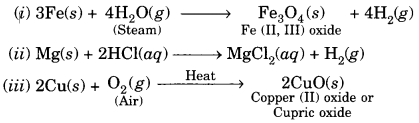Question 9.
fialance the following chemical equations and identify the type of chemical reaction.
(a) Mg (s) + Cl2 (g) → MgCl2 (s)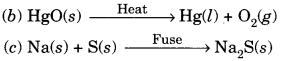(d) TiCl4 (l) + Mg (s) → Ti (s) + MgCl2 (s)
(e) CaO (s) + SiO2 (s) → CaSiO3 (s)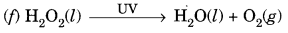[NCERT Exemplar]
The chemical equation in their balanced form may be written as follows:
(a) Mg (s) + Cl2 (g) → MgCl2 (s), Combination reaction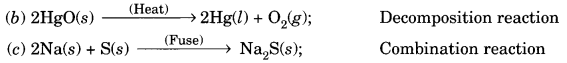(d) TiCl4 (l) + 2Mg (s) → Ti (s) + 2MgCl2 (s); Displacement reaction
(e) CaO (s) + SiO2 (s) → CaSiO3 (s); Combination reaction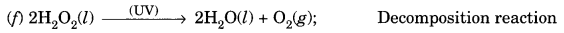Question 10.
A silver article generally turns black when kept in the open for a few days. The article when rubbed with toothpaste again starts shining.
(a) Why do silver articles turn black when kept in the open for a few days? Name the phenomenon involved.
(b) Name the black substance formed and give its chemical formula.   [NCERT Exemplar]
(a) Silver articles turn black when kept in the air for a few days because H2S gas present in the air attacks silver forming a coating of black silver sulphide. The phenomenon is called corrosion.

(b) Black substance formed is silver sulphide (Ag2S)
2Ag (s) + H2S (g) → Ag2S (s) + H2 (g).

Question 11.
Which among the following are physical or chemical changes?
(a) Evaporation of petrol
(b) Burning of Liquefied Petroleum Gas (LPG)
(c) Heating of an iron rod to red hot
(d) Curdling of milk
(e) Sublimation of solid ammonium chloride  [NCERT Exemplar]
(a) Physical change
(b) Chemical change
(c) Physical change
(d) Chemical change
(e) Physical change

### Extra Questions for Class 10 Science Chapter 1 Long Answer Type

Question 1.
Balance the following equations:
(a) Bacl2 + H2SO4 → BaSO4 + HCl
(b) CH4 + O2 → CO2 + H2O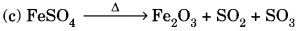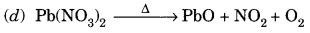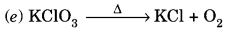(a) Bacl2 + H2SO4 → BaSO4 + 2HCl
(b) CH4 + 2O2 → CO2 + 2H2O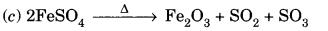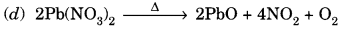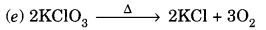Question 2.
On heating blue coloured powder of copper (II) nitrate in a boiling tube, copper oxide (black), oxygen gas and a brown gas X is formed.
(а) Write a balanced chemical equation of the reaction.
(b) Identify the brown gas X evolved.
(c) Identify the type of reaction.
(d) What could be the pH range of aqueous solution of the gas X?  [NCERT Exemplar]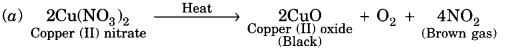(b) Brown gas X is nitrogen dioxide (NO2).
(c) It is a thermal decomposition reaction.
(d) The gas (NO2) is an oxide of a non-metal. Hence, its aqueous solution will be acidic, i.e., pH range would be between 0 and 7.

Question 3.
(A) Name the type of chemical reaction represented by the following equation: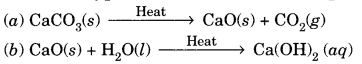(c) Zn(s) + H2SO4(ag) → ZnSO4(aq) + H2(g)
(B) “A solution of potassium chloride when mixed with silver nitrate solution, and an insoluble white substance is formed”.   [CBSE 2010, 2012]
(i) Translate the above statement into a chemical equation.
(ii) State two types for the classification of this reaction.
(A) (a) Decomposition reaction
(b) Combination reaction
(c) Displacement reaction.

(B) (i) KCl (aq) + AgNO3 (aq) → AgCl (s) + KNO3 (aq)
(ii) It is a double displacement reaction also called precipitation reaction.

Question 4.
What happens when zinc granules are treated with dilute solution of H2SO4, HCl, HNO3, NaCl and NaOH, also write the chemical equations if reaction occurs.  [NCERT Exemplar]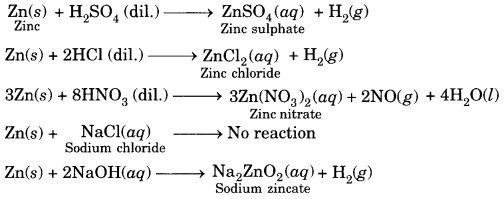Question 5.
(A) Write the balanced chemical equations for the following chemical reactions:
(i) Hydrogen + Chlorine → Hydrogen chloride
(iii) Zinc oxide + Carbon → Zinc + Carbon monoxide
(B) Write balanced chemical equations for the following reactions:
(a) Silver bromide on exposure to sunlight decomposes into silver and bromine.
(b) Sodium metal reacts with water to form sodium hydroxide and hydrogen gas.
(A) (i) H2 + Cl2 → 2HCl
(ii) Pb + CuCl2 → PbCl2 + Cu
(iii) ZnO + C → Zn + CO
(B)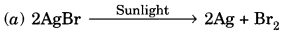(b) 2Na + 2H2O→ 2NaOH + H2

Question 6.
(a) Why cannot a chemical change be normally reversed?
(b) Why is it always essential to balance a chemical equation?
(c) What happens when CO2 gas is passed through lime water and why does it disappear on passing excess CO2?
(d) Can rusting of iron takes place in distilled water?
(a) In a chemical change some bonds are broken and some bonds are formed. The products are quite different from the reactants. Therefore, it normally can’t be reversed.
(b) A chemical equation has to be balanced to satisfy the law of conservation of mass.
(c) On passing CO2 gas through lime water, it turns milky due to formation of insoluble calcium carbonate which dissolves on passing excess CO2 due to formation of soluble calcium bicarbonate.
Ca(OH)2 + CO2(g) → CaCO3(s) + H2O(l)
CaCO3(s) + H2O(l) + CO2(g) → Ca(HCO3)2 (soluble)
(d) No

Question 7.
What happens when a piece of
(a) zinc metal is added to copper sulphate solution?
(b) aluminium metal is added to dilute hydrochloric acid?
(c) silver metal is added to copper sulphate solution?
Also, write the balanced chemical equation if the reaction occurs.  [NCERT Exemplar]
(a) Zinc is more reactive than copper. It displaces Cu from CuSO4 solution forming colourless zinc sulphate. Thus, blue colour of CuSO4 solution starts fading and ultimately blue colour disappears.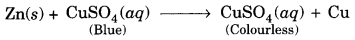(b) Aluminium reacts with dilute HCl acid forming AlCl3 along with evolution of bubbles of H2 gas.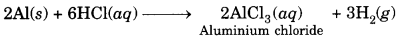(c) Silver is less reactive than copper. Hence, Ag cannot displace Cu from CuSO4 solution. Thus, no reaction occurs.

Question 8.
(A) A brown substance ‘X’ on heating in air forms a substance ‘Y’. When hydrogen gas is passed over heated ‘Y’, it again changes back into ‘X’.
(i) Name the substances X and Y.
(ii) Name the chemical processes occurring during both the changes.
(iii) Write the chemical equations.   [CBSE 2011]
(B) A metal is treated with dil. H2SO4. The gas evolved is collected by the method shown in the figure. Answer the following: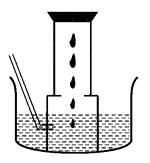(i) Name the gas.
(ii) Name the method of collection of the gas.
(iii) Is the gas soluble or insoluble in water?
(iv) Is the gas lighter or heavier than air?
(A) (i) The substance X is copper and Y is copper (II) oxide or CuO.
(ii) The process of change of X into Y is oxidation. The process of change of Y into X is reduction.
(iii) The chemical equations are: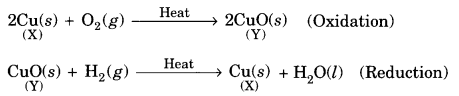(B) (i) The gas evolved is hydrogen.
(ii) The method of collection of the gas is the downward displacement of water.
(iii) The gas is insoluble in water. That is why, it can be collected over water.
(iv) The gas is lighter than air.

### Chemical Reactions and Equations HOTS Questions With Answers

Question 1.
The marble statues often slowly get corroded when kept in open for a long time. Assign a suitable explanation.
SO2, NO2 gases are released into the atmosphere from various sources. These dissolve in rain water to give acid which corrodes marble statues.
2SO2 + O2 → 2SO3
H2O + SO3 → H2SO4
2NO2 + H2O → 2HNO3
CaCO3 + H2SO4 → CaSO4 + H2O + CO2
CaCO3 + 2HNO3 → Ca(NO3)2 + H2O + CO2

Question 2.
Observe the following activity and identify the following: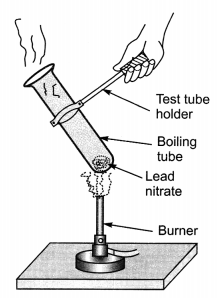(a) Type of chemical reaction.
(b) Write a chemical equation to represent the above change.
(c) Name the gas evolved in the above reaction.
(d) Do you observe any colour change in the above reaction? If yes, mention the colour change.
(e) Name the coloured substance formed.
(a) Decomposition reaction.
(b) 2Pb(NO3)2 (s) → 2PbO (s) + 4NO2 (g) + O2 (g)
(c) Reddish brown fumes of nitrogen dioxide along with oxygen gas is evolved.
(d) The colour of the substance changes from white to yellow due to formation of lead oxide.
(e) Lead oxide which is yellow coloured.

Question 3.
A substance X, which is an oxide of a group 2 element, is used intensively in the cement industry. This element is present in bones also. On treatment with water it forms a solution which turns red litmus blue. Identify X and also write the chemical reactions involved.   [NCERT Exemplar]
The above information suggests that the substance ‘A’ is oxide of the element calcium (Ca) which is present in group 2 of the periodic table. Calcium is also a constituent of our bones in the form of calcium phosphate. Calcium oxide (CaO) reacts with water to form calcium hydroxide (basic in nature). It forms a basic solution which turns red litmus blue.
CaO (s) + H2O (aq) → Ca(OH)2 (aq)

Question 4.
On adding a drop of barium chloride solution to an aqueous solution of sodium sulphite, a white precipitate is obtained.
(a) Write a balanced chemical equation of the reaction involved.
(b) What other name can be given to this precipitation reaction?
(c) On adding dilute hydrochloric acid to the reaction mixture, white precipitate disappears. Why? [NCERT Exemplar]
(a) A white precipitate of barium sulphite is formed when barium chloride is added to the solution of sodium sulphite.
BaCl2 (aq) + Na2SO3 (aq) → BaSO3 (s) + 2NaCl (aq)

(b) This precipitation reaction is also a double displacement reaction.

(c) Barium chloride, sulphur dioxide and water are formed when dilute hydrochloric acid is added to this solution of barium sulphate and sodium chloride. Since barium chloride is a soluble substance, thus white precipitate of barium sulphite disappears.
BaSO3 (s) + HCl (ag) → BaCl2 (ag) + SO2 (g) + H2O

Question 5.
A water insoluble substance ‘X’ on reacting with dilute H2SO4 released a colourless and odourless gas accompanied by brisk effervescence. When the gas was passed through water, the solution obtained turned blue litmus red. On bubbling the gas through lime water, it initially became milky and the milkiness disappeared when the gas was passed in excess. Identify the substance ‘X’ and write the chemical equations of the reaction involved.
The water insoluble substance ‘X’ is most probably the metal carbonate (CaCO3). The chemical reaction that were involved are given below.
CaCO3 (s) + H2SO4 (aq) → CaSO4 (ag) + H2O (aq) + CO2 (g)
Ca(OH)2 (aq) + CO2 (g) → CaCO3 (s) (milky) + H2O (l)
CaCO3 (s) + CO2 (g) + H2O (aq) → Ca (HCO3)2 (milkiness)

Question 6.
The given set up shows the electrolysis of water taking place.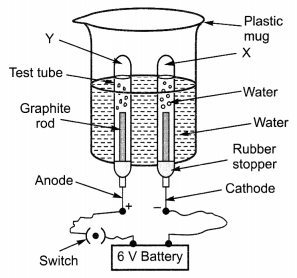(a) Identify the gases evolved at cathode and anode.
(b) Why is the amount of gas collected in one of the test tubes double the amount in the other? Name this gas.
(c) How will you test the evolved gases?
(a) The gases evolved at anode and cathode are oxygen and hydrogen.

(b) On electrolysis, water decomposes to form hydrogen and oxygen gas in the ratio of 2 : 1 by volume according to the equation given below: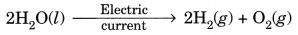The gas collected in double volume is hydrogen.

(c) Test for oxygen. If we bring a burning splinter near the mouth of test tube containing oxygen gas it burns more brightly.
Test for hydrogen. On bringing a burning splinter near the mouth of the test tube containing hydrogen, the gas burns with a pop sound.

Question 7.
A magnesium ribbon is burnt in oxygen to give a white compound X accompanied by emission of light. If the burning ribbon is now placed in an atmosphere of nitrogen, it continues to burn and forms a compound Y.
(a) Write the chemical formulae of X and Y.
(b) Write a balanced chemical equation, when X is dissolved in water.   [NCERT Exemplar]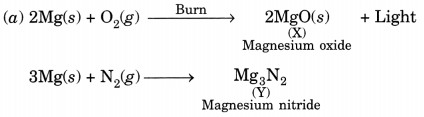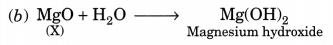Question 8.
You are provided with two containers made up of copper and aluminium. You are also provided with solutions of dilute HCl, dilute HNO3, ZnCl2 and H2O. In which of the above containers these solutions can be kept? [NCERT Exemplar]
(A) When solutions are kept in copper container
(a) Dilute HCl
Copper does not react with dilute HCl. Therefore, it can be kept.

(b) Dilute HNO3
Nitric acid acts as a strong oxidising agent and reacts with copper vessel, therefore cannot be kept.

(c) ZnCl2
Zinc is more reactive than copper (Cu) therefore, no displacement reaction occurs and hence can be kept.

(d) H2O
Copper does not react with water. Therefore, can be kept.

(B) When solutions are kept in aluminium containers
(a) Dilute HCl
Aluminium reacts with dilute HCl to form its salt and hydrogen is evolved. Therefore, cannot be kept.
2 Al + 6HCl → 2AlCl3 + 3H2

(b) Dilute HNO3
Aluminium gets oxidised by dilute HNO3 to form a layer of Al2O3 and can be kept.

(c) ZnCl2
Aluminium being more reactive than zinc can displace zinc ion from the solution. Therefore, the solution cannot be kept.
2Al + 3ZnCl2 → 2AlCl3 + 3Zn

(d) H2O
Aluminium does not react with cold or hot water. Therefore, water can be kept.
Aluminium is attacked by steam to form aluminium oxide and hydrogen.
2Al(s) + 3H2O(g) → Al2O3(S) + 3H2 (g)

### Extra Questions for Class 10 Science Chapter 1 Value Based

Question 1.
Rakesh wanted to give a coating of white wash on the walls of his house. He purchased quick lime (CaO) and dropped it in container of water and immediately applied the same on the walls. In this process, he spoiled his hands and even suffered minor bums. His friend Kapil advised him to keep the container overnight before applying a coating on the wall.
(i) What mistake was committed by the Rakesh?
(iii) Why did he suffer from minor bums?
(iii) How was Kapil’s advice useful?
(iv) What values are exhibited by Kapil?
(i) Rakesh should have waited for a few hours because when quick lime dissolves in water, slaked lime is formed and the process is highly exothermic (a lot of energy is released).

(ii) Because the reaction is exothermic and a lot of energy is released and container becomes hot so he suffered from minor burns.

(iii) Quick lime (CaO) reacts with water to form Ca(OH)2 which is known as slaked lime. The dissolution process is highly exothermic. So a large amount of heat is evolved. By keeping container overnight, the chemical reaction subsides and the heat dissipates. So the coating of slaked lime can be applied safely on the walls.

(iv) Knowledge of Chemistry, helpful and caring nature.

Question 2.
Palak is an eleven year old girl. She purchased a packet of potato chips and had a few from it more than a month back. She had kept away the open packet containing the remaining potato chips then. She wanted to eat the remaining potato chips now. Her elder sister Anjali found that the potato chips were giving out an unpleasant odour. When she put one of them in her mouth, she found that it had an unpleasant taste too. Anjali threw away the packet and did not allow Palak to eat the potato chips.
(a) What name is given to the condition in which potato chips kept in the open for a long time give out unpleasant smell and taste?
(b) Which chemical reaction is responsible for the spoilage of potato chips?
(c) Explain the reason for the unpleasant smell as well as unpleasant taste of the potato chips.
(d) Mention the values exhibited by Anjali.